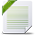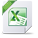# Decision Optimization

View Only

## Different results using Cplex in Matlab and Cplex in its own environment#### Alejandro Serrano FontovaFri December 10, 2021 07:08 AM• #### 1.  Different results using Cplex in Matlab and Cplex in its own environment

Posted Fri December 10, 2021 07:08 AM
Dear Network,

I'm trying to solve a mixed integer linear optimization program for network flow. If I launch the system in CPLEX (using the CPLEX STUDIO 12.10.0 environment) it gives different results than those obtained when using the same problem with exactly the same constraints but using the cplex solver in Matlab.

I've found out that the results are the same when power flow is positive. However, surprisingly, when the power flow is supposed to be negative (source is placed downstream the network) CPLEX does not accept negative values and asign zero to this values. However, when launching in Matlab environment using CPLEX as a solver, it gets the correct results (I attach the results of both cases; CPLEX itself and Matlab using CPLEX solver).

Could it be something related to tolerances? or another internal parameter of the CPLEX solver? This is driving me crazy as getting different results with same constraints and data is something unusual...

Alex

This is the code in CPLEX
- - - - - - -
using CPLEX;

// parameters

int n=5; // number of buses
int m=4; // number of lines
int y=1000; // large number
int z=350; // cost of LS
int t=1; // Rated voltage
float Vmax=1.05;// Max Voltage
float Vmin=0.95;// Min Voltage

range buses = 1..n;// length set of buses
range lines = 1..m;// length set of lines

float PLoad [buses] = [0,0.009,0.009,0.009,0.009];//Max Active Power of dispatchable DG
float QLoad [buses] = [0,0.006,0.006,0.006,0.006];//Max Reactive Power of dispatchable DG
float PDGmax[buses] = [0,0,0,0,0.036];//Active Power OF EACH BUS
float QDGmax[buses] = [0,0,0,0,0.024];//Reactive Power OF EACH BUS
float alpha [lines] = [0,1,1,1];//Alpha coefficient
float r [lines] = [0.093850842,0.025549741,0.044230064,0.044230064];//Lines resistance
float x [lines] = [0.084566834,0.029848586,0.058480517,0.058480517];//Lines reactance

// variables
dvar float+ Pl[lines];//Active power flow across lines
dvar float+ Ql[lines];//Reactive power flow across lines
dvar float+ PDG[buses];//Active power injected by DG
dvar float+ QDG[buses];//Reactive power injected by DG
dvar float+ Pls[buses];//Active powerload shedding flow across line
dvar float+ Qls[buses];//Reactive power load shedding flow across line
dvar float+ V[buses];//Reactive power load shedding flow across line
dvar float+ DELTAV[lines];//Reactive power load shedding flow across line
// model

minimize sum(i in buses) (Pls[i]+Qls[i])*z;

// constraints

subject to
{

forall (i in buses)

forall (i in buses)

forall (i in buses)
0<=PDG[i]<=PDGmax[i];//Limit for dispatachable DG Active power

forall (i in buses)
0<=QDG[i]<=QDGmax[i]; //Limit for non-dispatachable DG Reactive power

Pl - Pl==(PLoad-PDG-Pls);// ACTIVE power balance at each bus 2

Ql - Ql==(QLoad-QDG-Qls);// ACTIVE power balance at each bus 2

Pl - Pl==(PLoad-PDG-Pls);// ACTIVE power balance at each bus 3

Ql - Ql==(QLoad-QDG-Qls);// ACTIVE power balance at each bus 3

Pl - Pl==(PLoad-PDG-Pls);// ACTIVE power balance at each bus 4

Ql - Ql==(QLoad-QDG-Qls);// ACTIVE power balance at each bus 4

Pl==(PLoad-PDG-Pls);// ACTIVE power balance at each bus 5

Ql==(QLoad-QDG-Qls);// REACTIVE power balance at each bus 5

forall (i in lines)

DELTAV[i]==(Pl[i]*r[i]+Ql[i]*x[i])/t;//Voltage drop across DN lines

forall (i in lines)

(1-alpha[i])*-y<= (V[i]-V[i+1])- DELTAV[i]<=(1-alpha[i])*y;//Voltage drop constraint

forall (j in lines)

-1*alpha[j]<=Pl[j]<=1*alpha[j]; //active power limits

forall (j in lines)

-1*alpha[j]<= Ql[j]<=1*alpha[j];//reactive power limits

forall (i in buses)

Vmin<=V[i]<=Vmax;//Voltage bus limits
V==1;//Imposing voltage of the islanding root DG

}
- - - - - - -
This is the code in MATLAB.
clc;
clear;

%% 1.obtain data
mpc=IEEE4_bus;
PDGmax=mpc.PDGmax';
QDGmax=mpc.QDGmax';
Alpha=mpc.alpha';
r=mpc.r';
x=mpc.x';

%% 2.set parameters
nb=5; %number of buses
nl=4; %number of lines
U=1; %rated voltage
M=1000; %large number
Vmax=1.05; %maximum voltage
Vmin=0.95; %minimum voltage

%% save results
V_total=zeros(nb,1);
Vl_total=zeros(nl,1);
Pl_total=zeros(nl,1); %active power at each line
Ql_total=zeros(nl,1);
Pls_total=zeros(nb,1);
Qls_total=zeros(nb,1);
PDG_total=zeros(nb,1);
QDG_total=zeros(nb,1);
Cost_total=zeros(1,1);

%% variables
V=sdpvar(nb,1); %voltage at each bus
Vl=sdpvar(nl,1); %voltage drop at each line
Pl=sdpvar(nl,1); %active power at each line
Ql=sdpvar(nl,1); %reactive power at each line
PDG=sdpvar(nb,1); %active power injected by DG
QDG=sdpvar(nb,1); %reactive power injected by DG

%% set constraints
C=[];

%% active power load shedding constraints
for i=1:nb
end

%% reactive power load shedding constraints
for i=1:nb
end

%% DG active power constraints
for i=1:nb
C=[C,0<=PDG(i),PDG(i)<=PDGmax(i)];
end

%% DG reactive power constraints
for i=1:nb
C=[C,0<=QDG(i),QDG(i)<=QDGmax(i)];
end

%% active and reactive power constraints at each bus

%% voltage constraints
for i=1:nb
C=[C,Vmin<=V(i),V(i)<=Vmax];
end
V(5)=1;

%% voltage loss across each line
for l=1:nl
C=[C,Vl(l)==(Pl(l)*r(l)+Ql(l)*x(l))/U];
end

%% voltage loss constraints
for l=1:nl
C=[C,-M*(1-Alpha(l))<=V(l)-V(l+1)-Vl(l),V(l)-V(l+1)-Vl(l)<=M*(1-Alpha(l))];
end

%% active power constraints
for l=1:nl
C=[C,-1*Alpha(l)<=Pl(l),Pl(l)<=Alpha(l)];
end

%% active power constraints
for l=1:nl
C=[C,-1*Alpha(l)<=Ql(l),Ql(l)<=Alpha(l)];
end

%% obkective function
objective=sum(Pls.*m+Qls.*m);

%% solve
ops=sdpsettings('solver','cplex','verbose',0);
sol=optimize(C,objective,ops);

%% save data
Pls_total(:,1)=double(Pls);
Qls_total(:,1)=double(Qls);
Vl_total(:,1)=double(Vl);
Pl_total(:,1)=double(Pl);
Ql_total(:,1)=double(Ql);
PDG_total(:,1)=double(PDG);
QDG_total(:,1)=double(QDG);
V_total=double(V);
Cost_total=double(objective);

%% export data
% filename1='active power of load shedding.xlsx';
% writematrix(Pls_total,filename1);
% filename2='reactive power of load shedding.xlsx';
% writematrix(Qls_total,filename2);
% filename3='cost.xlsx';
% writematrix(Cost_total,filename3);
% filename4='voltage.xlsx';
% writematrix(V_total,filename4);
% filename5='active power flow.xlsx';
% writematrix(Pl_total,filename5);
% filename6='reactive power flow.xlsx';
% writematrix(Ql_total,filename6);

%% analysis error
if sol.problem==0
disp('successful solved');
else
disp('error');
yalmiperror(sol.problem)
end

------------------------------
Alejandro Serrano Fontova
------------------------------

#DecisionOptimization

Attachment(s)CPLEX RESULTS.ods   3 KB 1 version• #### 2.  RE: Different results using Cplex in Matlab and Cplex in its own environment

Posted Fri December 10, 2021 12:02 PM
It is unclear what you mean by "the power flow is supposed to be negative", but if you are referring to the values of some of the variables (PI, QI?) in the final solution, note that your OPL model declares those variables to be nonnegative (the "+" in "dvar float+").

------------------------------
Paul Rubin
Professor Emeritus
Michigan State University
------------------------------